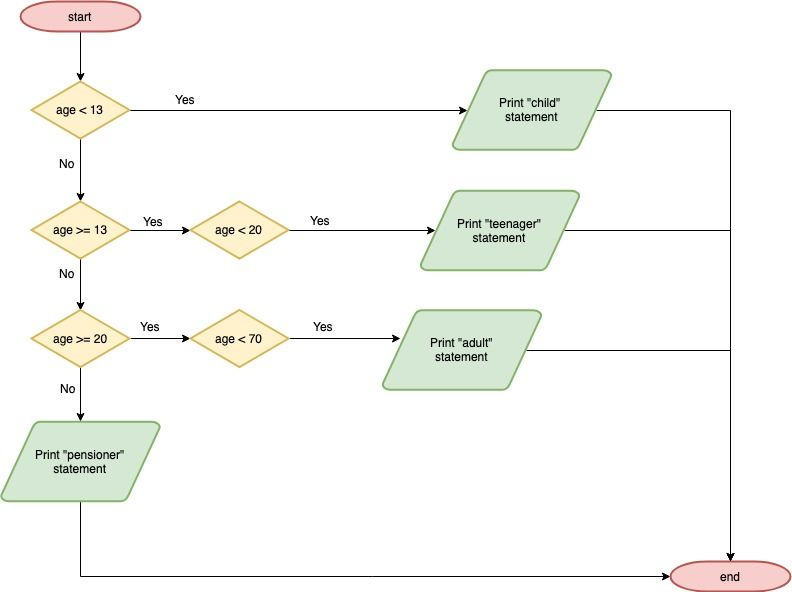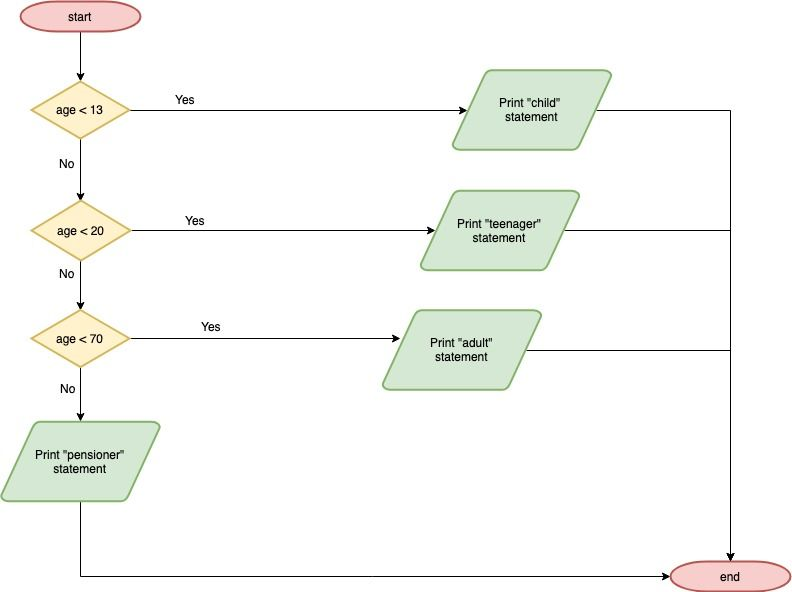# Conditionals in Go (challenge answer)At the end of the last article ( “Conditionals in Go” ), we said it was possible to optimise the `if/else if/else` statement further and gave you the challenge of figuring out how. If you haven’t attempted it; we’re about to give you the answer, so now’s a good time to go and try it.

For reference, here was the `if/else if/else` statement we said could be optimised:

``````if age < 13 {
log.Println("I am considered a child")
} else if age >= 13 && age < 20 {
log.Println("I am considered a teenager")
} else if age >= 20 && age < 70 {
} else {
log.Println("I am considered a pensioner")
}
``````

It can be optimised further because we’re still doing more checks than we need to. The following diagram shows the flow of this conditional statement:For example, we’re checking if `age` is less than thirteen ( `age < 13` ) and, if it is, we print the “child” statement and end the conditional statement. If it isn’t, we then check if age is greater than or equal to thirteen ( `age >= 13` ).

This extra step is unnecessary because we’ve already checked if age is less than thirteen and, if it isn’t, then it has to be equal to or greater than thirteen.

Knowing this, we can simplify the flow of our conditional statement to the following:And therefore our `if/else if/else` statement becomes:

``````if age < 13 {
log.Println("I am considered a child")
} else if age < 20 {
log.Println("I am considered a teenager")
} else if age < 70 {